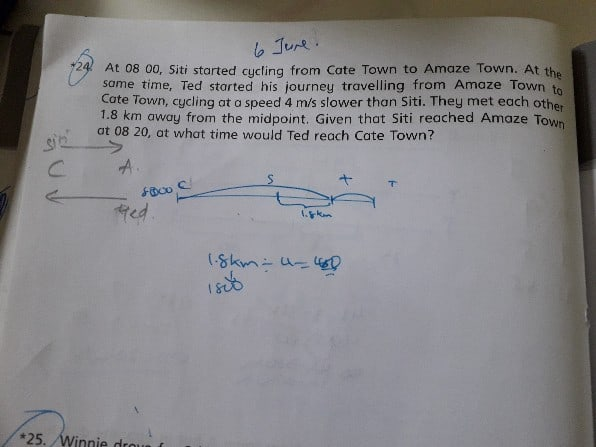# QuestionSiti covered 1.8*2=3.6km more than Ted did when they met each other because of the speed difference.

So it took 3.6km/4m/s=900s before they met each other.

lets say half of the distance is x, it took Siti 900s to meet Ted and 20*60=1200s to finish the travel

average speed for Siti was unchanged. So  (x+1800)/900=2x/(20×60) ⇒x=3600m

Siti’s Speed=(3600+1800)/900=6m/s, Ted’s speed=2m/s

time for Ted to reach =2*3600/2=3600s=60min, so he would reach there by 0900.

0 Replies 0 Likes